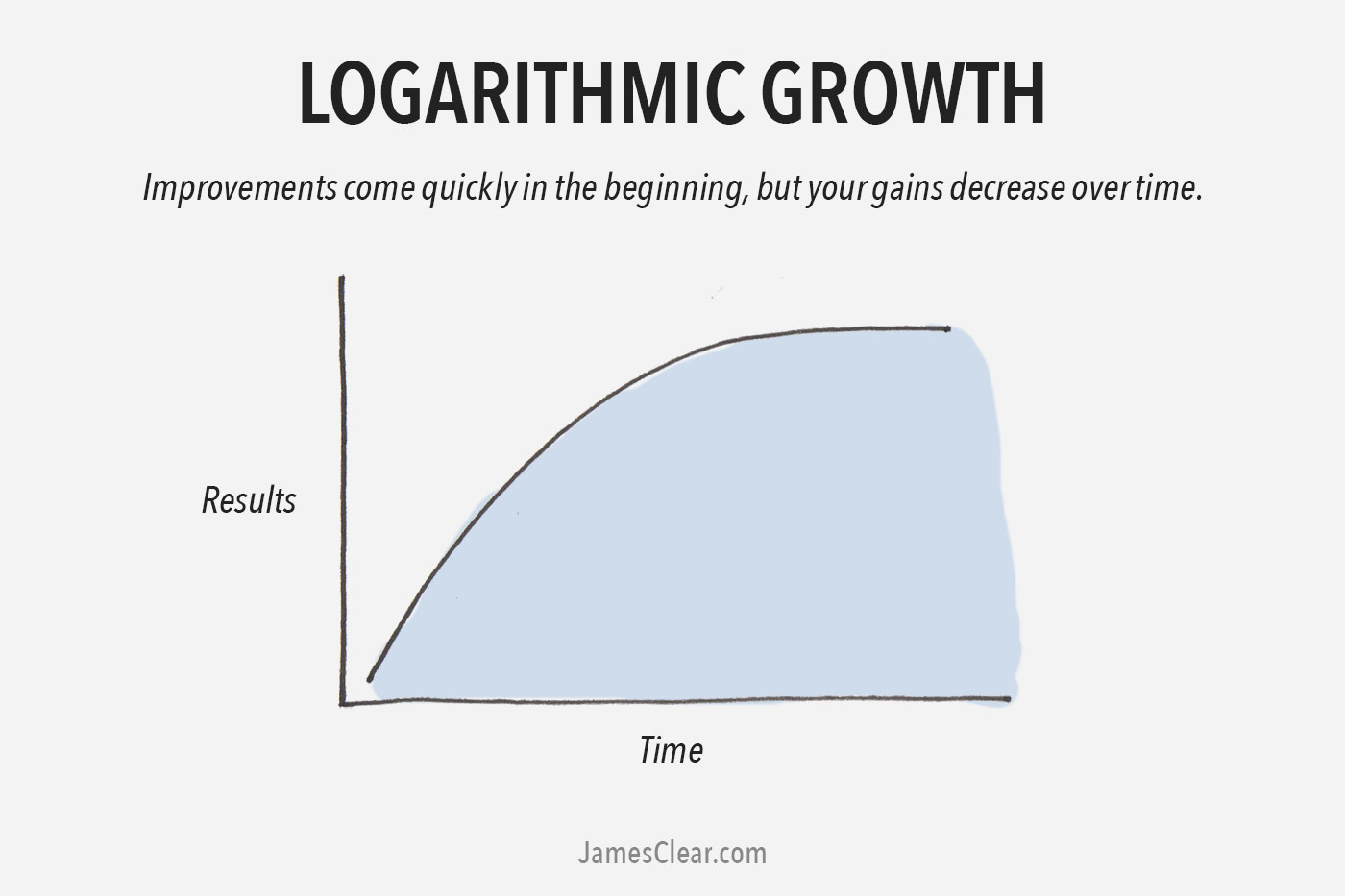## Friday, 21 February 2014

### Entropy and the Universe

Entropy and the UniverseMilky Way over Devil's Tower

Entropy is defined as the lack of order of predictability or the degree of randomness of a system. Over time all states as in temperature, pressure, and chemical potential will end in a state of thermodynamic equilibrium. This is called the second Law of Thermodynamics.

The entropy of the Universe continues to the maximum, as it rises over time. Time being measured in the billions of years. As entropy rises, the perception is that the amount of free energy and matter will diminish and will be unavailable for the entropy values to increase.

Hence a paradox arises. As work can no longer be done, then life as we know it may ceases to exist. At this point the Universe itself would cease to exist? This of course furthers the question of whether the Universe is an open system, and is the Universe in a state of equilibrium?

The equation of entropy for the Universe is;$\frac{dS}{dt} = \dot S_{i}$ with$\dot S_{i} \ge 0$

S is the entropy
t is time
Si is the sum of the rate of production of entropy

To plot a curve for the values of entropy and time a starting point is necessary, which brings into question whether entropy and time have a beginning, as in a Zero value. I have always contended that there has always been time and space in the Universe, so for lack of argument sake, the following will be that both values of entropy and time will approach zero.

The curve for time v.s entropy with time being the "x" axisFigure 1
This exponential curve illustrates entropy values increase over time, measured in billions of years. As entropy values increases, time over this period slows. This then follows with the entropy curve, depicted below.Figure 2
In the exponential curve above which is depicting the values of entropy as the "y" axis over time in the "x" axis, entropy increases in values until a point in the future where it's rate of incremental change slows, as the Universe's free matter and energy falls. This is what one would predict.

Both graphs predict that over time, both entropy and time approach zero.

Why would time slow as entropy values increase? Time slows to enable the curve in figure 2 to never approach zero This would mean that the rate of change of entropy throughout the course of time would remain more constant.

Time is also slowed, so the rising entropy values will continue, and are not predetermined by falling free energy and matter values in the Universe.

The most important explanation for time slowing is that it enables the thermodynamic state of the Universe to be close to a state of equilibrium from the Second Law of Thermodynamics. This state of equilibrium allows the Universe to exist forever. The Universe existing forever is a common believe that all of us hold.

Theorem;

The universe has existed and will exist forever:
Therefore time must slow